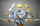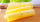# Fraction calculator

The calculator performs basic and advanced operations with fractions, expressions with fractions combined with integers, decimals, and mixed numbers. It also shows detailed step-by-step information about the fraction calculation procedure. Solve problems with two, three, or more fractions and numbers in one expression.

## Result:

### 3/4 * 2/7 = 3/14 ≅ 0.2142857

Spelled result in words is three fourteenths.

### How do you solve fractions step by step?

1. Multiple: 3/4 * 2/7 = 3 · 2/4 · 7 = 6/28 = 3 · 2/14 · 2 = 3/14
Multiply both numerators and denominators. Result fraction keep to lowest possible denominator GCD(6, 28) = 2. In the following intermediate step, cancel by a common factor of 2 gives 3/14.
In other words - three quarters multiplied by two sevenths = three fourteenths.

#### Rules for expressions with fractions:

Fractions - simply use a forward slash between the numerator and denominator, i.e., for five-hundredths, enter 5/100. If you are using mixed numbers, be sure to leave a single space between the whole and fraction part.
The slash separates the numerator (number above a fraction line) and denominator (number below).

Mixed numerals (mixed fractions or mixed numbers) write as integer separated by one space and fraction i.e., 1 2/3 (having the same sign). An example of a negative mixed fraction: -5 1/2.
Because slash is both signs for fraction line and division, we recommended use colon (:) as the operator of division fractions i.e., 1/2 : 3.

Decimals (decimal numbers) enter with a decimal point . and they are automatically converted to fractions - i.e. 1.45.

The colon : and slash / is the symbol of division. Can be used to divide mixed numbers 1 2/3 : 4 3/8 or can be used for write complex fractions i.e. 1/2 : 1/3.
An asterisk * or × is the symbol for multiplication.
Plus + is addition, minus sign - is subtraction and ()[] is mathematical parentheses.
The exponentiation/power symbol is ^ - for example: (7/8-4/5)^2 = (7/8-4/5)2

#### Examples:

subtracting fractions: 2/3 - 1/2
multiplying fractions: 7/8 * 3/9
dividing Fractions: 1/2 : 3/4
exponentiation of fraction: 3/5^3
fractional exponents: 16 ^ 1/2
adding fractions and mixed numbers: 8/5 + 6 2/7
dividing integer and fraction: 5 ÷ 1/2
complex fractions: 5/8 : 2 2/3
decimal to fraction: 0.625
Fraction to Decimal: 1/4
Fraction to Percent: 1/8 %
comparing fractions: 1/4 2/3
multiplying a fraction by a whole number: 6 * 3/4
square root of a fraction: sqrt(1/16)
reducing or simplifying the fraction (simplification) - dividing the numerator and denominator of a fraction by the same non-zero number - equivalent fraction: 4/22
expression with brackets: 1/3 * (1/2 - 3 3/8)
compound fraction: 3/4 of 5/7
fractions multiple: 2/3 of 3/5
divide to find the quotient: 3/5 ÷ 2/3

The calculator follows well-known rules for order of operations. The most common mnemonics for remembering this order of operations are:
PEMDAS - Parentheses, Exponents, Multiplication, Division, Addition, Subtraction.
BEDMAS - Brackets, Exponents, Division, Multiplication, Addition, Subtraction
BODMAS - Brackets, Of or Order, Division, Multiplication, Addition, Subtraction.
GEMDAS - Grouping Symbols - brackets (){}, Exponents, Multiplication, Division, Addition, Subtraction.
Be careful, always do multiplication and division before addition and subtraction. Some operators (+ and -) and (* and /) has the same priority and then must evaluate from left to right.

## Fractions in word problems:

• KingKing had four sons. First inherit 1/2, second 1/4, third 1/5 of property. What part of the property was left to the last of the brothers?
• Paper clipsMrs. Bright is organizing her office supplies. There are 5 open boxes of paper clips in her desk drawer. Each box has 1/2 of the paper clips remaining. How many boxes of paper clips are left?7 is added to the sum of 4/5 and 6/7
• Divide 13Divide. Simplify your answer and write as an improper fraction or whole number. 14÷8/3
• TeacherTeacher Rem bought 360 pieces of cupcakes for the outreach program of their school. 5/9 of the cupcakes were chocolate flavor and 1/4 wete pandan flavor and the rest were a vanilla flavor. How much more pandan flavor cupcakes than vanilla flavor?Max is working out 2/3+7/9. He says the answer is 9/12. What mistake have Max made?
• The recipeThe recipe they are following requires 7/8 cups of milk, Tom already put 3/8 cups of milk. How much milk should Lea add to follow the recipe?
• Obtuse angleWhich obtuse angle is creating clocks at 17:00?
• MichaelMichael had a bar of chocolate. He ate 1/2 of it and gave away 1/3. What fraction had he left?
• Unit rateFind unit rate: 6,840 customers in 45 days
• A shopkeeperA shopkeeper cuts a wheel of cheese into 10 equal wedges. A customer buys one-fifth of the wheel. How many wedges does the customer buy? Use the number line to help find the solution.
• Tallest and shortestJenn is the tallest on the team. He is 1 1/2 times as tall as the shortest girl whom is 4 1/4 feet tall. How tall is Jebb?Peter bought 1/2 a pound of chocolate at rocky mountain chocolate factory. Later he went to the sweet shoppie and he bought 6/9 of a pound more chocolate. How much chocolate did he buy that day?